Shubham Jain — December 4, 2017

## Introduction

Visualization is one of the most exciting parts of data science. Plotting huge amounts of data to unveil underlying relationships has its own fun.

Whether you’re identifying relationships between features or simply understanding the working of a model, visualizations are usually the best way to go about it. Visualizations also help explain your work to your customers and stakeholders.

Python provides a lot of libraries, specifically for plotting and visualization and I usually have a tough time picking out which one to use for my problem statement.

I recently come across with Altair, a visualization library in Python and I was amazed by its capabilities. It is a very user-friendly library which actually performs a lot of things with the minimal amount of code.

Please note that Altair is still in development phase, so things might change over time. We can still do a lot of exciting work on it and the future potential really excites me – hence this article!

So, let’s get started.

1. Overview of Altair
1. What is Altair?
2. Installation
2. Exploring a Real World Problem
3. Pros and Cons
4. End Notes

## 1. Overview of Altair

### 1.1 What is Altair?

Altair is a declarative statistical visualization library in Python, based on Vega-lite .

I am sure many of you would be asking these two questions by now:

• What is meant by declarative?
• Why is another library required for visualization?

By declarative, we mean that while plotting any chart, you only need to declare links between data columns to the encoding channels, such as x-axis, y-axis, colour, etc. and rest all of the plot details are handled automatically. Let’s understand it by an example.

Take a look at the plot below and above that is the code required for generating that plot in Altair.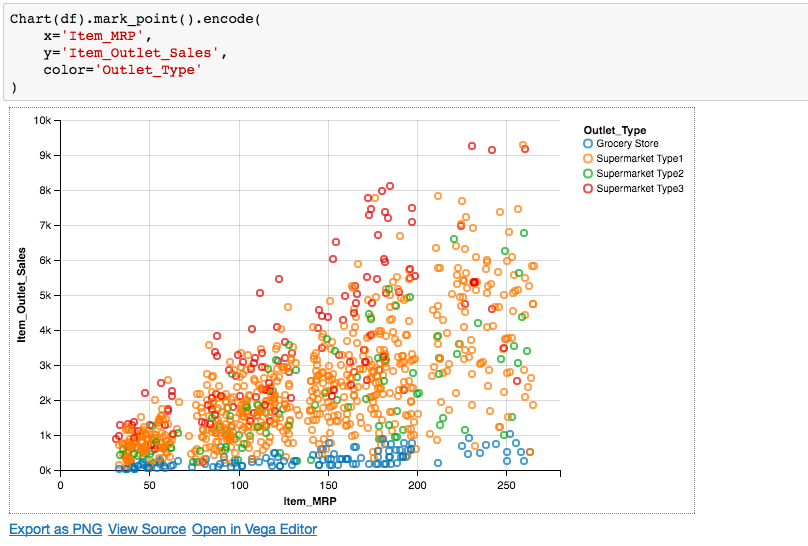So, if you notice, we just mentioned x,y and color and rest of the things like legend, axis labels, range etc. all are automatically set.

Now, take a look at the image below which is the matplotlib implementation of the same visualization we previously did with Altair.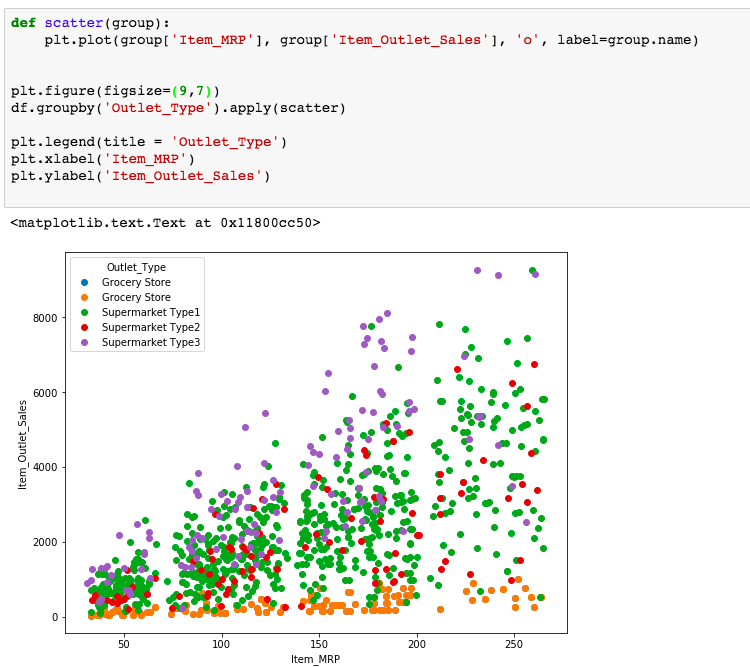Here, we need to explicitly use groupby function, define axis names, legends etc. which becomes a lot of extra work while you are doing an exploratory analysis.

Therefore being declarative makes Altair simple, friendly and consistent. It produces beautiful and effective visualizations with the minimal amount of code. Therefore you can spend more time understanding your data rather than spending your time on setting the legends, defining axes and so on.

I suppose by now you must have got the answer to the second question also.

### 1.2 Installation

Altair can be installed via conda as follows:

` conda install altair --channel conda-forge`

Or we can also install it via pip with the following:

``` pip install altair

Great, now you have Altair installed on your system. I always feel that the best way to learn a library is to practice it on a real-life problem. So, without any further delay, let us quickly begin!

## 2. Exploring a Real World Problem

In the data set, we have product wise Sales for Multiple outlets of a chain.  Let’s take a look at the dataset.

### 2.1 Understanding the dataset

```df = pd.read_csv('/Users/shubhamjain/Downloads/AV/Big Mart/train.csv')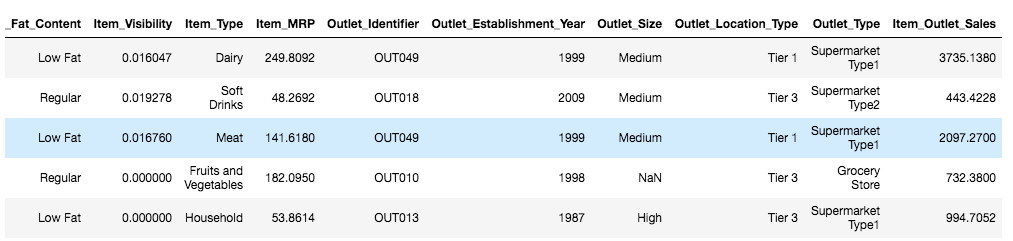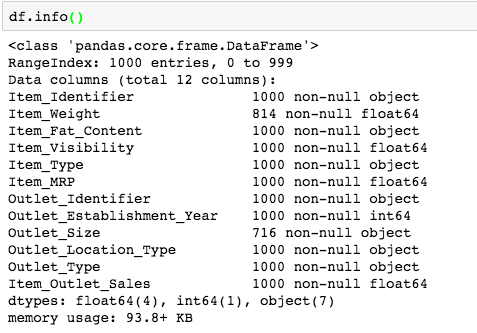The total data frame consists of 12 columns, out of which 7 are categorical and rest are numerical. Here, we have Item_Outlet_Sales as the target feature.

NOTE: I have only used the first 1000 rows, just to provide you with the sense of different plots using Altair.

### 2.2 Histograms

During the exploration journey, we generally start with the univariate analysis using histograms.

So, let’s start with the Item_Outlet_Sales feature, by plotting its histogram using Altair.

```Chart(df).mark_bar().encode(
X('Item_Outlet_Sales',bin=True),
Y('count(*):Q'),
)```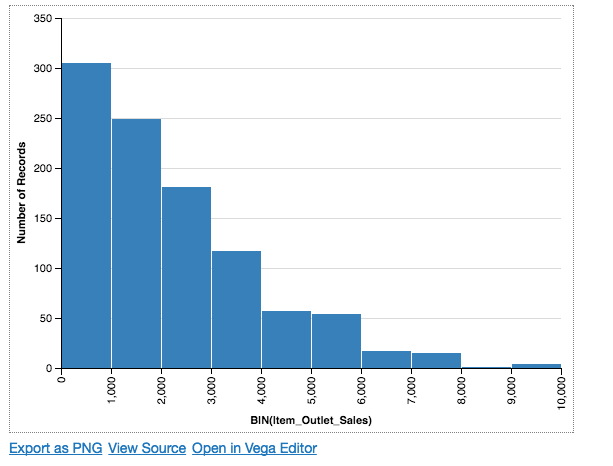To understand the code, let’s understand some basic terminologies, to begin with.

• Chart: It is the basic fundamental object in Altair, which takes data frame as the single argument.
• mark: It is an attribute to visualize your data points from the chart object. There are different forms of mark objects as shown below.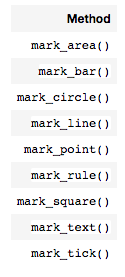Here, we have shown bar mark in order to visualization our data points as a bar chart.

• encode: It is a function which helps in mapping with different features from the data with the visualization. You can define features for the x-axis, y-axis, color etc.

Above defined are the common parameters which are used in every Altair visualization. Let’s take a look at some specific attributes which are used in the above code.

• bin: It is set TRUE to discretized a continuous feature, in order to produce a bar plot.
• count: It is a data transformation parameter, which is provided by Altair in order to customize our plots. We can also use other parameters like max, min, average, etc.
• Q: Here, Q denotes that the count will a quantitative feature. We can also use N for nominal data or O for ordinal data.

That was seriously very difficult to grasp after reading it for the first time. But, surely we will get hands-on it by the end of this article.

Okay, now if you look at the above chart, the label for x-axis is not defined properly by the Altair, and we wish to change it. You can do this by defining another parameter called axis.

```Chart(df).mark_bar().encode(
X('Item_Outlet_Sales',bin=True, axis= Axis(title='Item_Outlet_Sales (in bins)')),
Y('count(*):Q'),
)```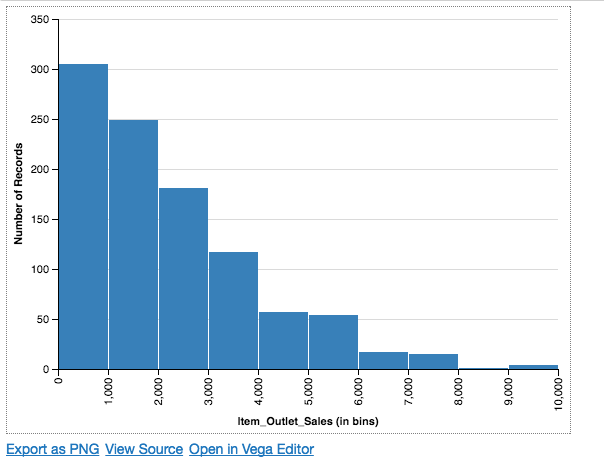It comes out to be a right-skewed distribution for the sales in our dataset. If I wish to do the same visualization using matplotlib, the implementation is shown below.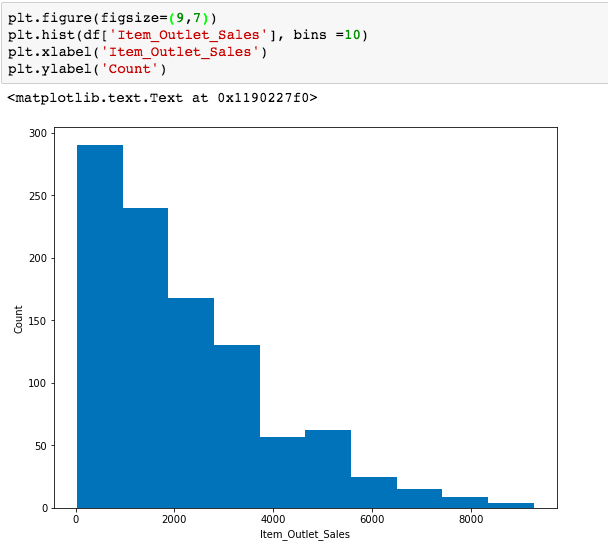You may consider the above code simple, but the visualization obtained from Altair is far more appealing than the one obtained from matplotlib.

We can also change the color of the histogram in Altair by simply adding a color attribute.

```Chart(df).mark_bar(color='lightblue').encode(
X('Item_Outlet_Sales',bin=True, axis= Axis(title='Item_Outlet_Sales (in bins)')),
Y('count(*):Q'),
)```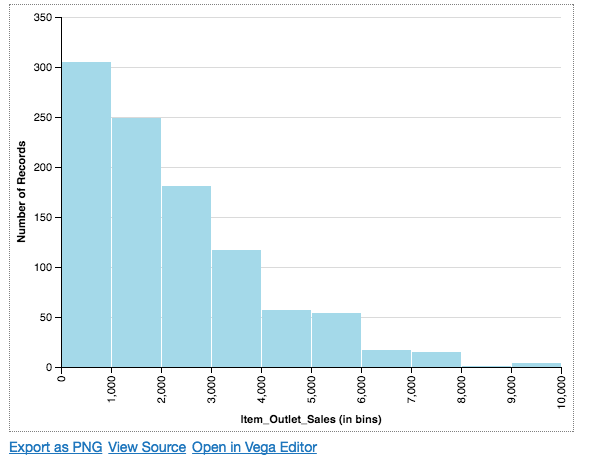### 2.3 Scatterplot

Now, let’s try to do some bivariate analysis using Altair.

Now, let us look at the relationship between MRP and Sales features.

```Chart(df).mark_point().encode(
x='Item_MRP',
y='Item_Outlet_Sales',
)```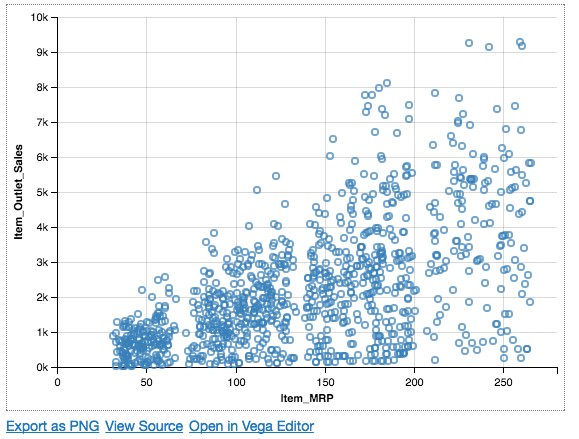We can clearly see a linear relationship between MRP and sales in the above plot. Let’s introduce some other encoding of Altair in order to draw more inferences from the visualizations.

```Chart(df).mark_point().encode(
x='Item_MRP',
y='Item_Outlet_Sales',
color='Outlet_Type'
)
```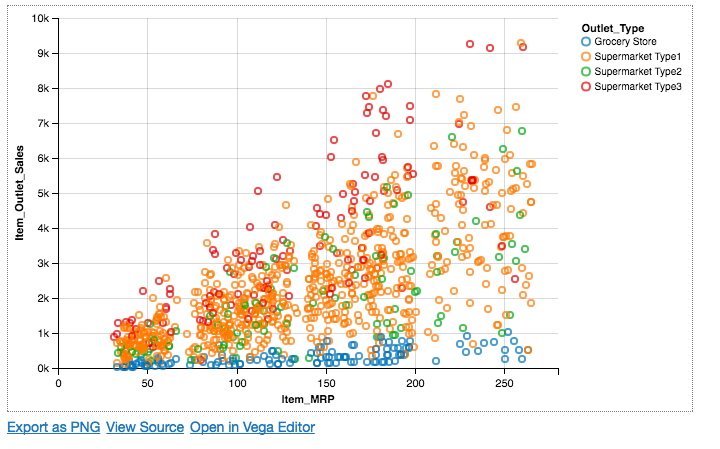Here, we have defined another encoding called color, which is used to differentiate the data points and helps us to understand the relationship better.

We can see that the sales for the grocery stores are pretty low as compared to sales in supermarkets, where supermarket type3 showing the highest sales.

```Chart(df).mark_point().encode(
x='Item_MRP',
y='Item_Outlet_Sales',
color='Item_Weight',
row='Outlet_Type'
).configure_cell(width=300, height=150)

```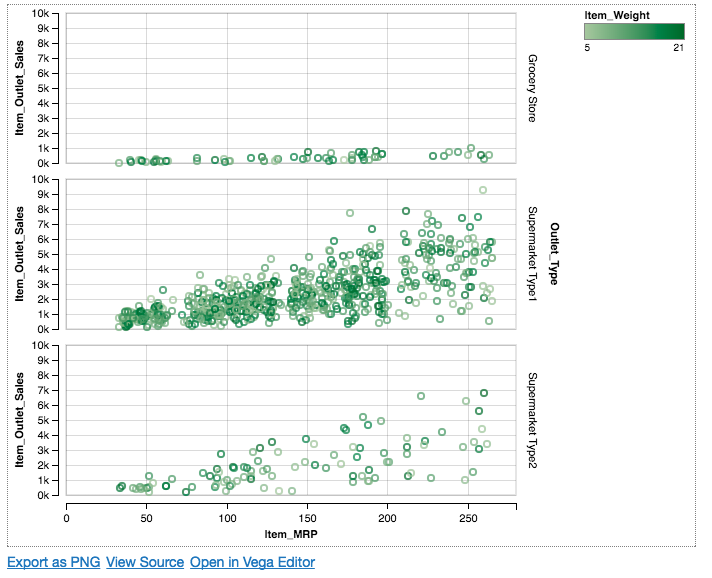So, we can now notice that items in supermarket type 1 have comparatively more weight than the grocery store and supermarket type 2.

These were different variates of scatter plot that can be drawn using the Altair. Let’s now look at some barplot charts to do some bivariate analysis.

### 2.4 Stacked Bar Plot

For this, let’s look at the relationship between Outlet_location_type and size of the outlet.

```Chart(df).mark_bar(stacked='normalize').encode(
Y('Outlet_Location_Type'),
X('count(*):Q', sort='descending'),
Color('Outlet_Size:N')
)
```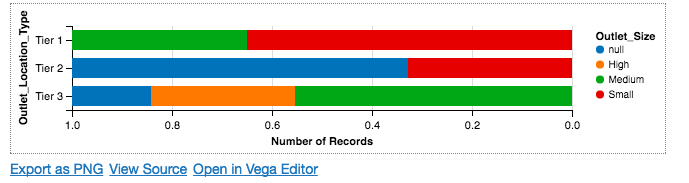Above drawn is the stacked bar plot, which denotes that the outlets in tier 2 cities only have outlets of small size, while only tier 3 cities have outlets which are high in size.

Notice that here we have defined the count aggregator in the X encoding, in order to produce a horizontal stack chart.

### 2.5 Line Chart

Now, let us look at the price of the item across the years. Generally, for the time series related visualizations, we prefer the use of line charts which is provided by Altair by using the line mark.

Altair supports date and discretization of dates when setting the type of it as temporal (T).

```Chart(df).mark_line().encode(
X('Outlet_Establishment_Year:T', timeUnit='year'),
Y('Item_MRP:Q', aggregate='mean'),
)```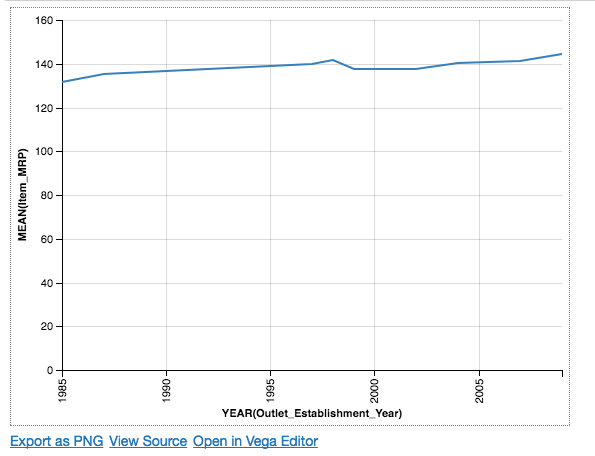Notice: Here, we have separately defined our transformation using the aggregate attribute.

### 2.6 Heatmap

Altair provides another type of chart known as heat map, which uses text mark attribute.

```Chart(df).mark_text(applyColorToBackground=True).encode(
Row('Outlet_Type:N'),
Column('Outlet_Size:N'),
Color('mean(Item_Outlet_Sales):Q', sort='descending'),
Text('mean(Item_Outlet_Sales):Q')
)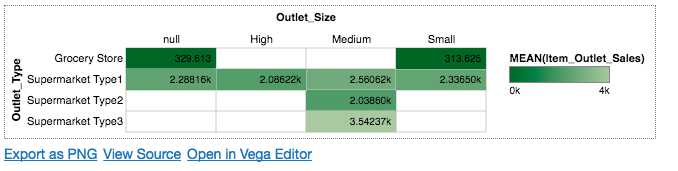```

Similarly, you can draw more patterns in data by this and you can also try some other data transformations and plots to find various trends and relationships between features.

Note that, Altair is currently in the development phase and sooner it will include more and better visualizations in the coming time.

## 3. Pros and Cons

Pros:

1. It is simple and produces more beautiful and effective visualizations.
2. The framework of code remains the same, i.e., just by changing the mark attribute you can produce different plots.
3. Data transformations like using count, min, max aggregator functions, is great to have in order to understand your data better.

Cons:

1. It doesn’t produce interactive visualizations like Bokeh or D3.js
2. Some of the major plots like boxplot are missing from Altair.

## 4. End Notes

I hope that you had a fun time learning this new library where you can do a lot of things with the minimal amount of code. So, try using it yourself on the dataset you are working on, and share your experience or doubts in the comment section below.

I would also recommend you to once the visit the gallery page of the Altair documentation for getting more idea about the type of visualizations possible using Altair.

Happy Exploring!###### Shubham Jain

I am currently pursing my B.Tech in Ceramic Engineering from IIT (B.H.U) Varanasi. I am an aspiring data scientist and a ML enthusiast. I am really passionate about changing the world by using artificial intelligence.

## 6 thoughts on "Introduction to Altair – A Declarative Visualization Library in Python"###### James Chibole says:December 04, 2017 at 11:48 am
Nice article on Altair and thank you for bringing up something that is still under production and explaining it extremely well. Reply###### Jarad says:December 05, 2017 at 3:39 am
piqued my interest but right before the first example you should have shown how you are importing altair. For example, altair.Chart, altair.X, and altair.Y are all classes. Sometimes it's nice to do detective work like this on our own as readers but when it's a tutorial-style post, it's not very smooth for the reader whose interested in getting started. My 2 cents. Reply###### Jarad says:December 05, 2017 at 3:58 am
Can one only render plots with: Jupyter Notebook, JupyterLab or nteract ? Reply###### Ram says:December 07, 2017 at 6:46 am
Hi, I am able to run the below code Chart(df).mark_bar().encode( X('Item_Outlet_Sales',bin=True), Y('count(*):Q'), ) But I am not able to see the output visualization . Could you please help me out with that Thanks Reply###### [email protected]says:December 07, 2017 at 9:08 am
Try installing these and run again. pip install jupyter pandas vega jupyter nbextension install --sys-prefix --py vega jupyter nbextension enable --sys-prefix --py vega Reference - https://altair-viz.github.io/installation.html#installation Reply###### SERGIO MARIN says:December 07, 2017 at 9:15 pm
A pity, I could not working Altair on Jupyter neither Spyder, It does nor work.... could you help me please...I really want to work with this package. Reply